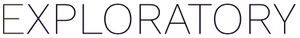# How to impute <NA> values in different categories?

#1

In this example dataframe:

The object is to replace the `<NA>` values with the “ReplacementRate” value from this dataframe:

What is the best way to do this? (I prefer to not use a join.)

For the first attempt, I used the `impute_na` function with the following statement

``````impute_na(OriginalRate, type = "value", val = Replacement\$ReplacementRate[Replacement\$RateType==RateType])
``````

``````'2. Create Calculation (Mutate)' step has an error. Error : Problem with `mutate()` input `OriginalRate`. x Can't recycle `..1` (size 10) to match `..2` (size 0). i Input `OriginalRate` is `impute_na(...)`.
``````

For the second attempt, I tried the `mutate` function:

with the following statement:

``````if_else(is.na(OriginalRate), Replacement\$ReplacementRate[Replacement\$RateType==RateType],OriginalRate)
``````

``````'2. Create Calculation (Mutate)' step has an error. Error : Problem with `mutate()` input `OriginalRate`. x `true` must be length 10 (length of `condition`) or one, not 0. i Input `OriginalRate` is `if_else(...)`.
``````

I suspect this has something to do with the scalar vs vector issue that @sugiaki nicely explained previously here:

but I still have no idea how to move past this!

Also, here another post that others might find helpful, where @Kei_Saito describes how to access the value in another dataframe:

`

#2

What is the best way to do this? (I prefer to not use a join.)

I think it would be easier to use the `Join` function, since it would require rewrite code when there are more categories to replace NA or some change…how about the following method?

It’s not flexible method, but I hope it helps.

``````# Write Custom R command in step
mutate(OriginRate_imputed =
case_when(RateType == "A" & is.na(OriginRate) ~ Replacement[Replacement\$RateType == 'A', 'ReplacementRate'],
RateType == "B" & is.na(OriginRate) ~ Replacement[Replacement\$RateType == 'B', 'ReplacementRate'],
TRUE ~ OriginRate)
)
``````

I think any of the following writing methods will work. The purpose of the script is the same.

``````# Get the ReplacementRate where RateType is A.
1. Replacement[Replacement\$RateType == 'A', 'ReplacementRate']
2. Replacement %>% filter(RateType == 'A') %>% select(ReplacementRate) %>% pull()
``````

The sample data was generated from the following code.

``````# Make `Original` dataframe
Original <-
data.frame(
ID = 0:9,
RateType = rep(c('B', 'A'), times = 5),
OriginRate = seq(0, 9.99, length.out = 10)
)
Original[4,3] <- NA
Original[9,3] <- NA

# Make `Replacement` dataframe
Replacement <-
data.frame(RateType = c('A','B'),
ReplacementRate = c(3.33, 8.88)
)
``````
#3

To me, using join() is a straightforward way to do it because you anyway need to refer to a different data frame. Also, on Exploratory Desktop, you can use UI for the join operation so you don’t need to maintain the code. Is there any particular reason you don’t want to use it?

#4

@sugiaki Thank you so much for your detailed answer. As you may have guessed, the inflexibility of coding it this way is a liability, so I think what you have proposed is a last resort.

@Kei_Saito regarding the `join` question, for my use case, I have two different categories of data that would need to be filtered before a join.

Let me see if I can explain my situation just a bit more clearly.

Similiar to what is shown below, there is an additional column:

that relates to two different dataframes:

Is there a good way to do this in a join?

FYI I tried creating two different branches, and that worked, but it became unwieldy. This is my effort to simplifi the code.

Thanks!

Update: thinking the `join` will work for me, and that filtering is not actually required!

Here is the code that worked:
`if_else(ReplacementNum == 1, impute_na(OriginalRate, type = "value", val = ReplacementRate1),impute_na(OriginalRate, type = "value", val = ReplacementRate2))`

Thank you both for helping me think through this.